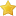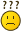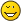Social Buttons

Thread Rating:
• 0 Vote(s) - 0 Average
• 1
• 2
• 3
• 4
• 5
 A token-based interpreter...
10-13-2018, 06:45 AM
Post: #1AurelAdministratorPosts: 171 Joined: Mar 2017 Reputation: 0
A token-based interpreter...
A token-based interpreter will have a loop and switch/select
somewhat like this:

Code:
```int *ip      'instruction pointer int  opcode  'instruction token As INT while (true) {    '// Read the next token from the instruction stream.    opcode = *ip    '// Advance to the next token in the stream.    ip++;    '// Decide what to do    select (opcode)       case tPrint: ' int tok=2            exec_Print()          ...                case else :            tExpr  'like LET tok=1            exec_Expr()          ...     end select            }```

Of before above step or block
we need to tokenize source code into token array
and this should be very clear step according to chosen syntax.

Attached File(s) Image(s)10-18-2018, 06:22 PM
Post: #2AurelAdministratorPosts: 171 Joined: Mar 2017 Reputation: 0
RE: A token-based interpreter...
In fact there is no many such a examples on internet
most of them build AST...
i ask myself why ? if there is no significant speed-up10-23-2018, 04:47 AM
Post: #3AurelAdministratorPosts: 171 Joined: Mar 2017 Reputation: 0
RE: A token interpreter (N.A.F.I.)
Code:
```' New Aurel Fancy Interpreter -> N.A.F.I. ' this is some tips  about nafi structre '////////////////////////////////////////////// example : a = 5 \$b = "Aurel" TOKEN-- --TOKENTYPE---- --varID---- --------numVAR ----------- ----- strVAR ---------- ________+_______________+___________+__________________________+________________​_______ a         nVar          1               ID,value ________________________________________________________________________________​_______ \$b        sVar          2                                    ID,value[Aurel] '---------------------------------------------------------------------------------------```
11-15-2018, 08:23 AM
Post: #4AurelAdministratorPosts: 171 Joined: Mar 2017 Reputation: 0
RE: A token-based interpreter...
from stackoverflow: C expr eval

#include <stdio.h>
#include <stdlib.h>

double expression(void);

double vars; // variables

char get(void) { char c = getchar(); return c; } // get one byte
char peek(void) { char c = getchar(); ungetc(c, stdin); return c; } // peek at next byte
double number(void) { double d; scanf("%lf", &d); return d; } // read one double

void expect(char c) { // expect char c from stream
char d = get();
if (c != d) {
fprintf(stderr, "Error: Expected %c but got %c.\n", c, d);
}
}

double factor(void) { // read a factor
double f;
char c = peek();
if (c == '(') { // an expression inside parantesis?
expect('(');
f = expression();
expect(')');
} else if (c >= 'A' && c <= 'Z') { // a variable ?
expect©;
f = vars[c - 'A'];
} else { // or, a number?
f = number();
}
return f;
}

double term(void) { // read a term
double t = factor();
while (peek() == '*' || peek() == '/') { // * or / more factors
char c = get();
if (c == '*') {
t = t * factor();
} else {
t = t / factor();
}
}
return t;
}

double expression(void) { // read an expression
double e = term();
while (peek() == '+' || peek() == '-') { // + or - more terms
char c = get();
if (c == '+') {
e = e + term();
} else {
e = e - term();
}
}
return e;
}

double statement(void) { // read a statement
double ret;
char c = peek();
if (c >= 'A' && c <= 'Z') { // variable ?
expect©;
if (peek() == '=') { // assignment ?
expect('=');
double val = expression();
vars[c - 'A'] = val;
ret = val;
} else {
ungetc(c, stdin);
ret = expression();
}
} else {
ret = expression();
}
expect('\n');
return ret;
}

int main(void) {
printf("> "); fflush(stdout);

for (;{
double v = statement();
printf(" = %lf\n> ", v); fflush(stdout);
}
return EXIT_SUCCESS;
}
02-09-2019, 09:08 AM
Post: #5AurelAdministratorPosts: 171 Joined: Mar 2017 Reputation: 0
RE: A token-based interpreter...
this one is from Jimage interpreter
need connection with ANIscript because require tokenizer.

Code:
```\$ Filename "RDescent.exe" ' o2 include "RTL32.inc" include "awinh037.inc" #lookahead 'Globals.................................................................. string token :  int tc int tokPLUS = 1, tokMINUS = 2 , tokMULTI = 3 ,tokDIVIDE = 4 int tokPOW = 5 , tokMOD = 7 , tokNUM = 8, tokVAR = 9, tokSTR = 10 int tokLPAREN = 11 , tokRPAREN = 12 , tokEOL = 13 , tokCOLON = 14 'open window............................................................. INT win,wx=0,wy=0,ww=600,wh=400,wstyle = WS_MINMAXSIZE INT button0,b0ID=100, lvControl,lvID=1000 win = SetWindow("RECURSIVE DESCENT EXPR-EVALUATOR",wx,wy,ww,wh,0,wstyle) 'token field - tokenized code------------------------------------------- string tokList[] = {"2","+","3","*","4"}    int tokType[] = { 8,  1 , 8 , 3 , 8 } InterpretTokens() '---------------------------------------------------------------------- 'message loop Wait() '---------------------------------------------------------------------- 'func main Function WndProc (sys hwnd,wmsg,wparam,lparam) as sys callback SELECT hwnd     CASE win         Select wmsg             CASE WM_CLOSE             CloseWindow(win)             EndProgram()         End Select END SELECT Return DefWindowProc(hwnd,wMsg,wParam,lParam) END FUNCTION '---- user functions ............................................ Function getToken() as int         tc++ ' token_count + 1         token = tokList[tc] : INT tok = tokType[tc]          print "TOKEN: " + token      return tok End function '............................................................... Function InterpretTokens() int tok,nextTok tok = getToken() : nextTok = tokType[tc+1]     Select tok         CASE tokNUM  '8-number          If nextTok = tokPLUS Or nextTok = tokMINUS Or nextTok = tokMULTI Or nextTok = tokDIVIDE              EvalExpr()         End if     End Select End function '------------------------------------------------------------ Function EvalExpr() float result : string res result = getExpression() print "RESULT: " + str(result) 'show result End Function ',,,,,,,,,,,,,,,,,,,,,,,,,,,,,,,,,,,,,,,,,,,,,,,,,,,,,,,,,,,,,,, function getExpression() as float         float value = getTerm()         int ntok         While 1             ntok = tokType[tc+1]             If ntok = tokPLUS      '"+"                 getToken()                 value = value + getTerm()             ElseIf ntok = tokMINUS '"-"                 getToken()                 value = value - getTerm()             Else             Exit while             End If         Wend         Return value end function ',,,,,,,,,,,,,,,,,,,,,,,,,,,,,,,,,,,,,,,,,,,,,,,,,,,,,,,,,,,,,,,,,,,,, function getTerm() as float         float value = getFactor()         int ntok         ntok = tokType[tc+1]             select ntok                 Case tokMULTI '"*"                 getToken(): value = value * getFactor()                 Case "/"                 getToken(): value = value / getFactor()                 'Case "%": getToken(): value = value % getFactor() '; break;                 'Case "&": getToken(): value = int(value) & int(getFactor())                 'Case "|": getToken(): value = int(value) | int(getFactor())                 Case "^"                 getToken(): value = value ^ int(getFactor())                                end select                  Return value end function ',,,,,,,,,,,,,,,,,,,,,,,,,,,,,,,,,,,,,,,,,,,,,,,,,,,,,,,,,,,,,,,,,,,,,,,,     function getFactor() as float {         double value = 0.0;         Variable v = null;         getToken();         switch (token) {             Case NUMBER:                 value = tokenValue;                 Break;             Case NUMERIC_FUNCTION:                 value = func.getFunctionValue(pgm.table[tokenAddress].type);                 Break;             Case STRING_FUNCTION:                 String str = func.getStringFunction(pgm.table[tokenAddress].type);                 value = Tools.parseDouble(str);                 If ("NaN".equals(str))                     value = Double.NaN;                 Else If (Double.isNaN(value))                     error("Numeric value expected");                 Break;             Case USER_FUNCTION:                 v = runUserFunction();                 If (v==null)                     error("No return value");                 If (done)                     value = 0;                 Else {                     If (v.getString()!=null)                         error("Numeric return value expected");                     Else                         value = v.getValue();                 }                 Break;             Case TRUE: value = 1.0; break;             Case FALSE: value = 0.0; break;             Case PI: value = Math.PI; break;             Case NaN: value = Double.NaN; break;             Case WORD:                 v = lookupVariable();                 If (v==null)                     Return 0.0;                 int Next = nextToken();                 If (Next=='[') {                     v = getArrayElement(v);                     value = v.getValue();                     Next = nextToken();                 } Else If (Next=='.') {                     value = getArrayLength(v);                     Next = nextToken();                 } Else {                     If (prefixValue!=0 && !checkingType) {                         v.setValue(v.getValue()+prefixValue);                         prefixValue = 0;                     }                     value = v.getValue();                 }                 If (!(Next==PLUS_PLUS || Next==MINUS_MINUS))                     Break;                 getToken();                 If (token==PLUS_PLUS)                     v.setValue(v.getValue()+(checkingType?0:1));                 Else                     v.setValue(v.getValue()-(checkingType?0:1));                 Break;             Case (int)'(':                 value = getLogicalExpression();                 getRightParen();                 Break;             Case PLUS_PLUS:                 prefixValue = 1;                 value = getFactor();                 Break;             Case MINUS_MINUS:                 prefixValue = -1;                 value = getFactor();                 Break;             Case '!':                 value = getFactor();                 If (value==0.0 || value==1.0) {                     value = value==0.0?1.0:0.0;                 } Else                     error("Boolean expected");                 Break;             Case '-':                 value = -getFactor();                 Break;             Case '~':                 value = ~(int)getFactor();                 Break;             Default:                 error("Number or numeric function expected");         }         '// IJ.log("getFactor: "+value+" "+pgm.decodeToken(preToken,0));         Return value;     }```

Attached File(s) Image(s)02-16-2019, 09:12 AM
Post: #6AurelAdministratorPosts: 171 Joined: Mar 2017 Reputation: 0
RE: A token-based interpreter...
and here is unfinished microB tokenizer

Code:
```'microB tokenizer int tkNULL=0,tkPLUS=1,tkMINUS=2,tkMULTI=3,tkDIVIDE=4 int tkCOLON=5,tkCOMMA=6,tkLPAREN=7,tkRPAREN=8,tkLBRACKET=9,tkRBRACKET=10 int tkPRINT=11,tkDOT=12,tkLINE=13,tkCIRCLE=14 ,tkEOL = 20 string tokList : int typList   'token/type arrays int start , p = 1 ,start = p ,tp ,n      'init string code,ch,tk ,crlf=chr(13)+chr(10),bf code = "var1 + 2.5 " ' test or load_src? sub tokenizer(src as string) as int 'ch = mid(src,p,1) : print "CH:" + ch' get first char while p <= len(src)              ch = mid(src,p,1)                    'get char If asc(ch)=32 : p++ : end if             'skip blank space              If (asc(ch)>96 and asc(ch)<123)          ' [a-z]        print "CH2:" + ch : p--    while (asc(ch)>96 and asc(ch)<123) or (asc(ch)>47 and asc(ch)<58) ' [a-z0-9]*        p++:ch = mid(src,p,1) : print "AZ:" + ch        tk =tk+ch      wend       print "TOK-AZ:" + tk        tp++ : tokList[tp] = tk : tk="" :p++              'return IDENT; Elseif (asc(ch)>47 and asc(ch)<58)                    ' [0-9]         p--     while (asc(ch)>47 and asc(ch)<58) or (asc(ch)=46)  '[0-9[0.0]]*         p++ : ch = mid(src,p,1):tk = tk + ch     wend        tp++ : tokList[tp] = tk : tk="":p++        'return NUMBER; Elseif asc(ch)=43                                     ' [ + ]        tk = ch : tp++ : tokList[tp] = tk : tk="" :p++  ' set_token      'elseif... End if wend return tp end sub 'call tokenizer..tested(ident,numbers) int tn: tn = tokenizer(code) : print "number of tokens:" + str(tn) for n = 1 to tn : bf = bf + tokList[n] + crlf : next n print  bf```
03-19-2019, 06:43 AM
Post: #7AurelAdministratorPosts: 171 Joined: Mar 2017 Reputation: 0
RE: A token-based interpreter...
..tiny tokenizer for microB(i hope that this schould be name)anyway it sems so far that tokenize properly:

ident( keywords,variables{a..z, a..z09) low case only(for now)
operators (+ , - , * , / , = , < , > )
quoted string(literal) : "quoted"
parens (brackets) - () , []
special - comma,colon,,,

code:::

Code:
```'microB tokenizer by Aurel 18.3.2019 int tkNULL=0,tkPLUS=1,tkMINUS=2,tkMULTI=3,tkDIVIDE=4 int tkCOLON=5,tkCOMMA=6,tkLPAREN=7,tkRPAREN=8,tkLBRACKET=9,tkRBRACKET=10 int tkPRINT=11,tkDOT=12,tkLINE=13,tkCIRCLE=14 ,tkEOL = 20 string tokList : int typList   'token/type arrays int start , p = 1 ,start = p ,tp ,n      'init string code,ch,tk ,crlf=chr(13)+chr(10),bf '-------------------------------------------------------------------- code = "let func(a,b): var1+ 0.5*7: str s="+ chr(34)+ "micro" + chr(34)  ' test or load_src? '-------------------------------------------------------------------- sub tokenizer(src as string) as int 'ch = mid(src,p,1) : print "CH:" + ch' get first char while p <= len(src)     ' print "P:" + str(p)              ch = mid(src,p,1)                   'get char If asc(ch)=32 then p=p+1 : end if             'skip blank space[ ] If asc(ch)=9  then p=p+1 : end if             'skip TAB [    ] '-------------------------------------------------------- If asc(ch)=34 ' if char is QUOTE " ' print mid(src,p+1,1) p++ :  ch = mid(src,p,1) : tk=ch : p++        'skip quote :add ch TO tk buffer: p+1     while asc(ch) <> 34 'and mid(src,p+1,1)<> chr(34)                ch = mid(src,p,1) : if asc(ch)= 34 then exit while         tk=tk+ch : p++         IF ch = chr(10): print "Unclosed Quote! Exit...": exit sub : end if     wend     tp++ : tokList[tp] = tk : tk="":ch="": p++  'add quoted string to token list End if '-------------------------------------------------------             If (asc(ch)>96 and asc(ch)<123)          ' [a-z]    while (asc(ch)>96 and asc(ch)<123) or (asc(ch)>47 and asc(ch)<58) ' [a-z0-9]*          'print "AZ:" + ch          tk=tk+ch : p++ : ch = mid(src,p,1)    wend       'print "TOK-AZ:" + tk + " PAZ:" + p        tp++ : tokList[tp] = tk : tk="":ch=""              'return IDENT; End If '-------------------------------------------------------------- 'While (Asc(Look) > 47 And Asc(Look) < 58) Or Asc(Look) = 46' If (asc(ch)>47 and asc(ch)<58)                    ' [0-9.]     while (asc(ch)>47 AND asc(ch)<58) OR asc(ch)=46  '[0-9[0.0]]*         tk=tk+ch :p++         ch = mid(src,p,1)     wend         'print "Pnum:" + str(p)        tp++ : tokList[tp] = tk : tk="":ch=""        'return NUMBER; End if '--------------------------------------------------- If asc(ch)=43 : tp++ : tokList[tp] = ch : ch="" : p++ : End if  ' + plus If asc(ch)=45 : tp++ : tokList[tp] = ch : ch="" : p++ : End if  ' - minus If asc(ch)=42 : tp++ : tokList[tp] = ch : ch="" : p++ : End if  ' * multiply If asc(ch)=47 : tp++ : tokList[tp] = ch : ch="" : p++ : End if  ' / divide If asc(ch)=40 : tp++ : tokList[tp] = ch : ch="" : p++ : End if  ' ( Lparen If asc(ch)=41 : tp++ : tokList[tp] = ch : ch="" : p++ : End if  ' ) Rparen If asc(ch)=44 : tp++ : tokList[tp] = ch : ch="" : p++ : End if  ' , comma If asc(ch)=58 : tp++ : tokList[tp] = ch : ch="" : p++ : End if  ' : colon If asc(ch)=60 : tp++ : tokList[tp] = ch : ch="" : p++ : End if  ' < less If asc(ch)=61 : tp++ : tokList[tp] = ch : ch="" : p++ : End if  ' = equal If asc(ch)=62 : tp++ : tokList[tp] = ch : ch="" : p++ : End if  ' > more(greater) 'elseif... 'End if IF ASC(ch)>125: print "Unknown token!-[" +ch +" ]-Exit...": RETURN 0: END IF wend return tp end sub 'call tokenizer..tested(ident,numbers) int tn: tn = tokenizer(code) : print "number of tokens:" + str(tn) for n = 1 to tn : bf = bf + tokList[n] + crlf : next n print  bf```

Attached File(s) Image(s)« Next Oldest | Next Newest »

Forum Jump:

User(s) browsing this thread: